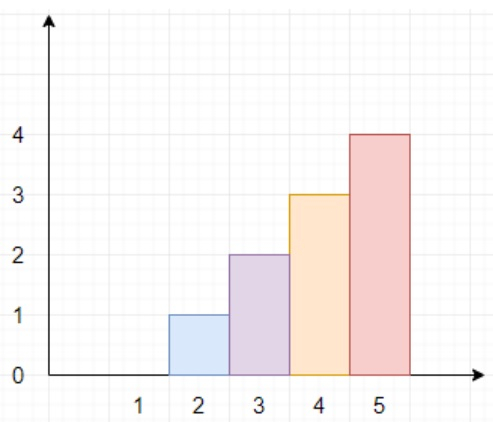# Program to find maximum building height in Python

Suppose we have a value n and another list of pairs called restrictions. We want to build n new buildings in a city. But there are few restrictions. We can built in a line and buildings are labeled from 1 to n. The restrictions has two parameters, so restrictions[i] = (id_i, max_height_i) indicates id_i must have height less than or equal to max_height_i. The city restrictions on the heights of the new buildings are as follows −

• The height of each building must be 0 or positive values.

• First building height must be 0.

• The difference between any two adjacent buildings height cannot exceed 1.

We have to find the maximum possible height of the tallest building.

So, if the input is like n = 5, restrictions = [[2,1],[4,3]], then the output will be 4 because we have to find maximum possible height, so it can be 4 as shown in the diagram.To solve this, we will follow these steps −

• if restrictions is empty, then

• return n-1

• resi := sort the list restrictions based on id

• k := 0

• idx := 1

• for each re in resi, do

• re := minimum of re and (k+re-idx)

• k := re

• idx := re

• k := max height of last element in resi

• idx := id of last element in resi

• reverse the list resi

• for each re in resi from first item onwards, do

• re := minimum of re and (k-re+idx)

• k := re

• idx := re

• reverse the list resi

• f := 0

• idx := 1

• res := 0

• for each re in resi, do

• ff := minimum of (f+re-idx) and re

• res := maximum of res and quotient of (re - idx + f + ff)/2

• idx := re

• f := ff

• return maximum of (f+n-idx) and res

## Example

Let us see the following implementation to get better understanding

def solve(n, restrictions):
if not restrictions:
return n-1
resi = sorted(restrictions, key = lambda x:x)

k = 0
idx = 1
for re in resi:
re = min(re, k+re-idx)
k = re
idx = re
k = resi[-1]
idx = resi[-1]
resi.reverse()
for re in resi[1:]:
re = min(re, k-re+idx)
k = re
idx = re
resi.reverse()

f = 0
idx = 1
res = 0
for re in resi:
ff = min(f+re-idx, re)
res = max(res, (re - idx + f + ff) // 2)
idx = re
f = ff
return max(f+n-idx,res)

n = 5
restrictions = [[2,1],[4,3]]
print(solve(n, restrictions))

## Input

5, [[2,1],[4,3]]


## Output

4